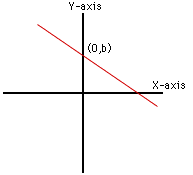Sender: Don
Subject: math problem

What is the Y-intercept for the problem:Y= -2(x+2) + 9

Hi Don

 The Y-intercept of a curve is the Y-coordinate of the point where the curve crosses the Y-axis. In the diagram on the right it is the number b. Since the X-coordinate of this point is zero you can find b by substituting x=0 into the equation. For your equation, Y = -2(x+2) + 9, substituting x=0 gives b = -2(0+2) + 9 = -2(2) + 9 = -4 + 9 = 5 Cheers, HarleyGo to Math Central

To return to the previous page use your browser's back button.